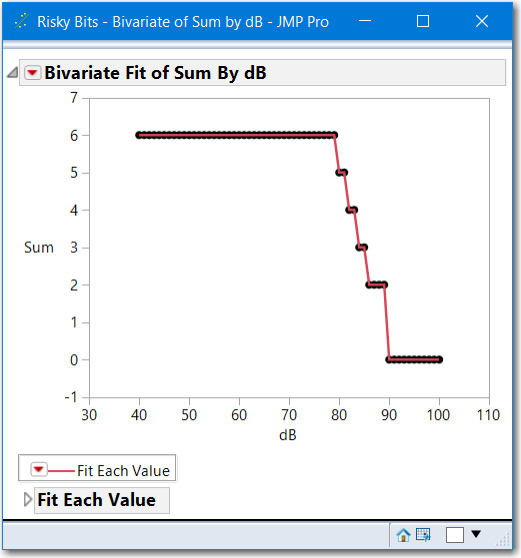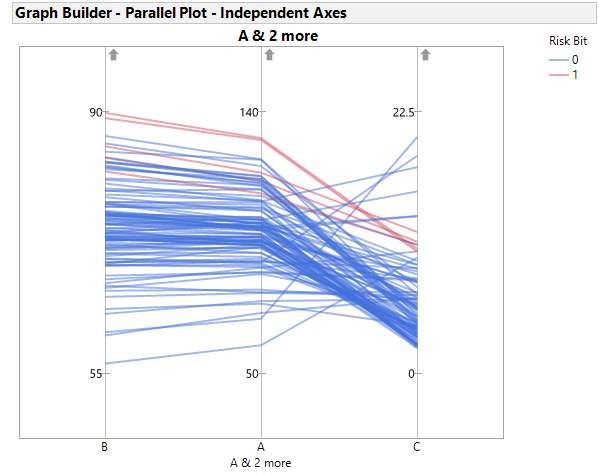Choose Language Hide Translation Bar
Highlighted

## Need help understanding parameters and table variables

Hi!

I have a practical problem that I think I could solve using some kind of parameter or table variable.

Let's say I have multiple processes, each having a row. Which are characterized by few parameters, A, B, C... Those are columns.

Let's say for each parameter I have a threshold after which it is considered risky. So I created columns bit-A, bit-B etc. If parameter is grater than some number, I give it bit "1", if it's below - I give it bit "0". Then I do logical  AND for all the bits and if it's "1" (all parameters are in "risky" zone) I call the whole process "risky". Let's say final risky bit is saved in column bit-Risky.

So, I'm sure about thresholds on A and C, but I'm not sure about threshold on B. So I want to see how number of risky processes depends on threshold for B (let's call it dB).

So, how do I plot Sum(bit-Risky) vs. dB?

1 ACCEPTED SOLUTION

Accepted Solutions
Highlighted

## Re: Need help understanding parameters and table variables

@miguello There might be a method to do this with linked profilers, but I think you might want to do this with a script.

Attached is a table, my representation of what you described. The table has a script to aggregate (find the sum) for various scenarios, various thresholds and store them in a table, then plot the Sum vs. dB.This could be done with an interactive window and made quite fancy.  But this is just a simple script with hard-coded names, and thershold ranges.

In the past, I have experienced similar requests for results of complex simulators, like semiconductor simulators.  Instead of counting, I think there is more insight by doing some analysis on the relationships of pass/fail.  Also attached to the table is a parallel plot (see below) script that can provide some insight to the variables.  Here a Local filter could be added and ranges of A, B and C can be selected.2 REPLIES 2
Highlighted

## Re: Need help understanding parameters and table variables

@miguello There might be a method to do this with linked profilers, but I think you might want to do this with a script.

Attached is a table, my representation of what you described. The table has a script to aggregate (find the sum) for various scenarios, various thresholds and store them in a table, then plot the Sum vs. dB.This could be done with an interactive window and made quite fancy.  But this is just a simple script with hard-coded names, and thershold ranges.

In the past, I have experienced similar requests for results of complex simulators, like semiconductor simulators.  Instead of counting, I think there is more insight by doing some analysis on the relationships of pass/fail.  Also attached to the table is a parallel plot (see below) script that can provide some insight to the variables.  Here a Local filter could be added and ranges of A, B and C can be selected.Highlighted

## Re: Need help understanding parameters and table variables

Thanks! This definitely works!
However, my specific problem is a little bit more complex than I initially described.
Each process has around hyndred test locations. So, just getting a sum of bits wouldn't do it.
Plus the conditions on thresholds might be complex as well - like, range, text comparison etc.
The way I eventually did it - I was just iterating through thresholds in the script. Luckily, all the bit columns were updated on threshold change. All I needed to do is to get a summary table and then I could get my risky bits as matrix and sum them - as you suggested.
Article Labels

There are no labels assigned to this post.